The rod

The rod has a length of 90cm. Half is painted blue, the third is red and the rest is yellow. How long is the yellow part of the rod?

Result

z =  15 cm

Solution:

90 = 90/2 + 90/3 + z

6z = 90

z = 15

Calculated by our simple equation calculator.

Leave us a comment of this math problem and its solution (i.e. if it is still somewhat unclear...):Be the first to comment!To solve this verbal math problem are needed these knowledge from mathematics:

Do you have a linear equation or system of equations and looking for its solution? Or do you have quadratic equation? Do you want to convert length units?

Next similar math problems:

1. Wood 11Father has 12 1/5 meters long wood. Then I cut the wood into two pieces. One part is 7 3/5 meters long. Calculate the length of the other wood?
2. Mr. TolentinoMr. Tolentino own a piece of land, it measures 86.12 meters long 68.293 meters width. How much longer is the length than the width?
3. Class alphabet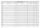All 29 pupils in the class are written in a class by alphabet. The number of pupils enrolled before Paul is three times higher than the number of pupils behind him. Calculate how many pupils are enrolled after Paul.
4. Bean bag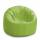A student tossed a bean bag. It landed 216 inches away. How many yards are equal to 216 inches?
5. Drive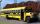At the bus stop 8 people take out and 10 people take into bus. Next stop 6 take out and 5 take in. On the third stop 6 take out and 3 take in. The bus traveled further with 39 people. How many passengers were originally at the bus?
6. Forest nurseryIn the forest nursery after winter, they found that 1/10 stems died out of them. For them, they land 193 new spruces. How many spruces are in the forest nursery?
7. Simple equation 8Solve the following equation: 36=-(1+7x)-6(-7-x)
8. Eq1Solve equation: 4(a-3)=3(2a-5)
9. Flood waterFlood waters in some US village meant that the homes had to evacuate 364 people. 50 of them stayed at elementary schools, 59 them slept with their friends and others went to relatives. How many people have gone to relatives?
10. Addition of Roman numbersAdded together and write as decimal number: LXVII + MLXIV
11. If-then equationIf 5x - 17 = -x + 7, then x =
12. Six te 2If 3t-7=5t, then 6t=
13. I think numberI think number.When I add 841 to it and subtract 157, I get a number that is 22 greater than 996. What number I thinking?
14. Minutes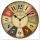Determine the difference in minutes: T1 = 2 3/20 h T2 = 2.3 h
15. Father and son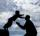Father is 44 years old, his son 16 years. Determine how many years ago was the father five times older than the son.
16. Bus tickets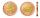Bus ticket for a trip from Prague to Paris cost 2180Kč. A return ticket costs 3930Kč. How much money will save a family of four to go to Paris and back when they purchase a return tickets?
17. BenchesThe park has 64 benches. Occupied are by 18 more than empty. How many benches are occupied and empty ?